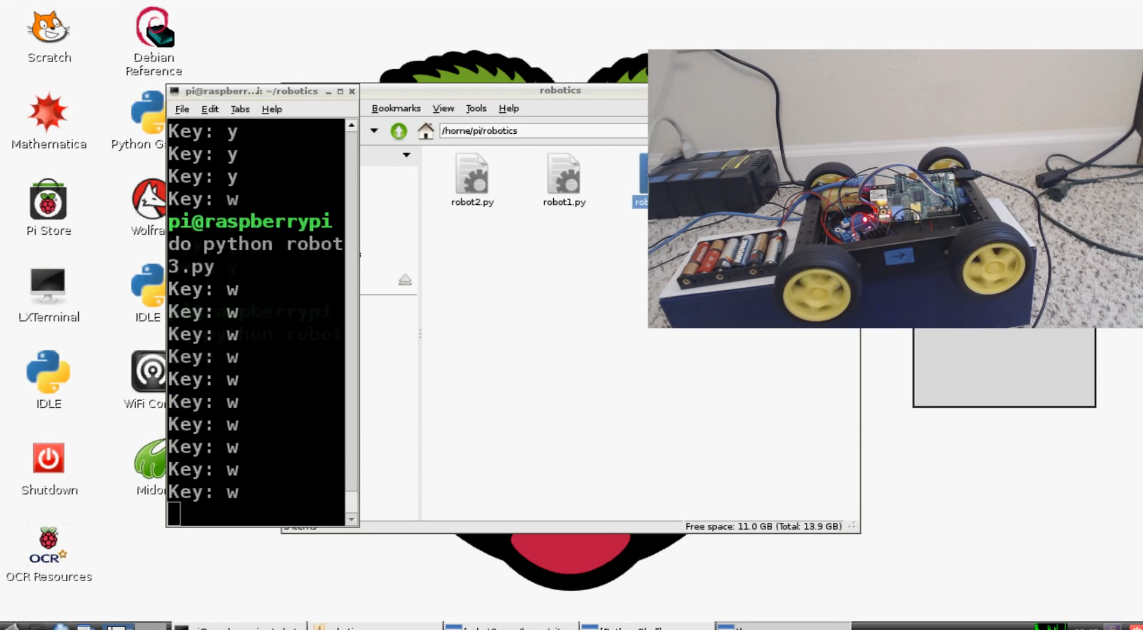## User Control for Remote Control Raspberry Pi Car

```import RPi.GPIO as gpio
import sys
import time
####
import Tkinter as tk

def init():
gpio.setmode(gpio.BOARD)
gpio.setup(7, gpio.OUT)
gpio.setup(11, gpio.OUT)
gpio.setup(13, gpio.OUT)
gpio.setup(15, gpio.OUT)
#gpio.output(7, True)
#gpio.output(11, True)

def forward(tf):
#init()
gpio.output(13, True)
gpio.output(15, False)

gpio.output(7, False)
gpio.output(11, True)
time.sleep(tf)
gpio.cleanup()

def reverse(tf):
#init()
gpio.output(13, False)
gpio.output(15, True)

gpio.output(7, True)
gpio.output(11, False)
time.sleep(tf)
gpio.cleanup()

def pivot_left(tf):
#init()
gpio.output(13, True)
gpio.output(15, False)
gpio.output(7, True)
gpio.output(11, False)
time.sleep(tf)
gpio.cleanup()

def pivot_right(tf):
#init()
gpio.output(13, False)
gpio.output(15, True)
gpio.output(7, False)
gpio.output(11, True)
time.sleep(tf)
gpio.cleanup()

def turn_left(tf):
#init()
gpio.output(7, True)
gpio.output(11, True)
gpio.output(13, True)
gpio.output(15, False)
time.sleep(tf)
gpio.cleanup()

def turn_right(tf):
#init()
gpio.output(7, False)
gpio.output(13, False)
gpio.output(15, False)
gpio.output(11, True)

time.sleep(tf)
gpio.cleanup()

def key_input(event):
init()
print 'Key:', event.char
key_press = event.char
sleep_time = 0.030

if key_press.lower() == 'w':
forward(sleep_time)
elif key_press.lower() == 's':
reverse(sleep_time)
elif key_press.lower() == 'a':
turn_left(sleep_time)
elif key_press.lower() == 'd':
turn_right(sleep_time)
elif key_press.lower() =='q':
pivot_left(sleep_time)
elif key_press.lower() == 'e':
pivot_right(sleep_time)

root = tk.Tk()
root.bind('<KeyPress>', key_input)
root.mainloop()

```

Alright, there is a bit more to this script. I will explain what is happening here, though you can also watch the video for a more in-depth explanation.

First, we needed some way to create a key-logger. The only way I know how is a non-headless way, which is okay, but not ideal. To do it, we're using event-handling built into Tkinter.

Note: import syntax for tkinter is different in Python 2 and Python 3. This is the Python 2 syntax here.

Our Tkinter application is just a simple window that records key presses. It's basically a key-logger.

Since our keylogger application is looping, we no longer need to call the init() function at the beginning of each of the functions.

Our major addition is the key_input() function, which is mainly our tkinter rules. The idea here is to print out what key is being pressed, after running an init(). Then, we define key_press as the key that is pressed.

Next, we have a sleep_time. I chose 0.030 because that allowed me to press and hold the keys for a while, release, and things would stop. Depending on a lot of small variables, your ideal time may vary. Try a few, and make sure you eliminate the lag.

Now we just define rules for each of the possible, acceptable, key-presses.

The next tutorial:• Robotics with the Raspberry Pi

• Programming GPIO example

• Running GPIO

• Building Autonomous / RC car intro

• Supplies needed

• Motor Control

• Connecting the four motors

• Forward and Reverse

• Turning

• Pivoting

• User Control
• Remotely controlling the car

• Adding a distance sensor (HC-SR04)

• Programming with the distance sensor

• Autopilot and/or auto-correct

• Autonomous Beginnings

• Testing Autonomous Code

• Streaming video example one

• Less latency streaming option